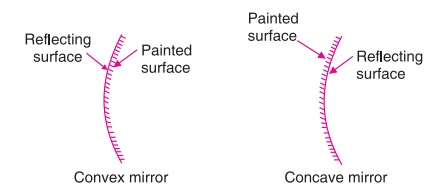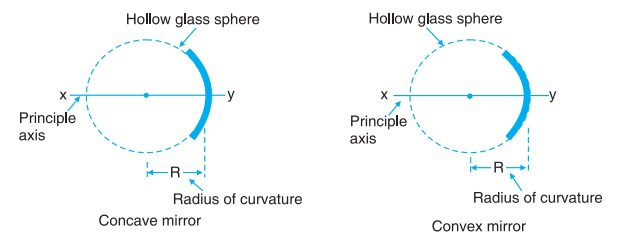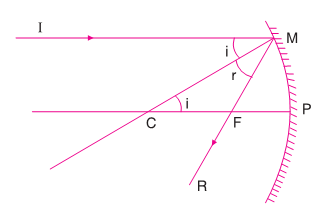# Reflection at Spherical Mirrors

A spherical mirror is a section of a hollow sphere whose inner or outer surface is polished. Thus, there are mainly two types of spherical mirrors (i) convex mirror and (ii) concave mirror.

1. Convex mirror: It is a mirror in which the reflection takes place from the bulging surface. Inner side is painted and reflected surface is polished to make the surface smooth.

2. Concave mirror: It is a mirror in which the reflection takes place from the cave side surface. Outer side is painted and the inner or cave side surface is polished to make the reflected surface smooth.### Important Terms

1. Pole (P): It is the mid point of the spherical mirror. Point P is the pole.

2. Centre of curvature (C): It is the centre of a hollow sphere of which the spherical mirror is a part. It can be determined by finding the point of intersection of two normal drawn at the spherical surface of the mirror. The point C is the centre of curvature.

3. Radius of curvature (R): It is the distance between the pole and centre of curvature of the mirror. CF is the radius of curvature.

4. Principal axis: It is an imaginary line joining the pole to the centre of curvature. Extended line CP is the principal axis.

5. Principal focus (F): The rays of light parallel and closed to the principal axis of the mirror after reflection, either pass through a point (in concave mirror) or appear to be coming from a point (in convex mirror) on the principal axis. This point is called principal focus of the mirror. Point F is the principal focus.

6. Focal length (f): It is the distance between the pole and the principal focus of the mirror. PF is the focal length.### Relation Between f and R

Consider the reflection of light of ray IM at M at a concave mirror. CM is the normal drawn at the surface which passes through centre of curvature and MF is the reflected ray which passes through the focal point.Angle of incidence and reflection are equal.

∠i = ∠r

In ΔCMF, MF = CF

For small aperture of the mirror,

MF = PF

PC = PF + CF = PF + PF = 2PF

R = 2f

where R = radius of curvature and f is the focal length of the mirror.